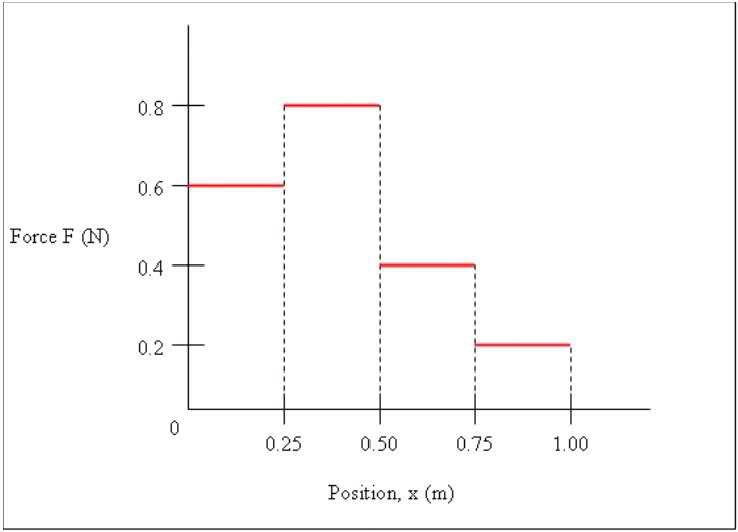# Problem: Work done by a Variable Force

## Homework Statement

An object is acted on by the force shown below. What is the final position of the object if
its initial position is x = 0.40m and the work done on it is equal to
(a) 0.21 J
(b) (b) -0.19 J.## Homework Equations

W = F1x1 + F2(x2 - x1)

## The Attempt at a Solution

a) W= F(x2-x1) = 0.8N* (x2- 0.40m)

but i DON'T get the answer correctly.

Last edited:

CompuChip
Homework Helper
How do you determine the work done from the graph you have given? Does that correspond to the formula you gave?

tiny-tim
Homework Helper
Welcome to PF!

a) W= F(x2-x1) = 0.8N* (x2- 0.40m)

but i DON'T get the answer correctly.

Hi lucky_star! Welcome to PF!Why have you used only one value of F?

F has different values as x changes.

Oh, I made a mistake there. if initial position is 0.40m, the force is 0.8N. I am given W= 0.21J, So I will have:

0.21J= 0.8N*0.40m + 0.8N*(x2 - 0.40m)
0.21J= 0.8x2 - 0.32
x2= 0.66m

That's all I have. However, the correct answer that I have been provided is 0.90m. I really don't know how to solve this problem. Please give a hint!

Hi lucky_star! Welcome to PF!Why have you used only one value of F?

F has different values as x changes.

I know the initial position is .40m, but I don't know which are the corresponding forces :(

tiny-tim
Homework Helper
if initial position is 0.40m, the force is 0.8N. I am given W= 0.21J, So I will have:

0.21J= 0.8N*0.40m + 0.8N*(x2 - 0.40m)
0.21J= 0.8x2 - 0.32
x2= 0.66m

Noooo …Look at the graph!

The force is 0.8N only for x ≤ 0.50m.

Try again.Noooo …Look at the graph!

The force is 0.8N only for x ≤ 0.50m.

Try again.AHHH! I Think I understand the problem.

W= 0.8N *(0.5m-0.4m) + 0.4N *(0.75m-0.5m) + 0.2 *(x2-0.75m)
0.21J = 0.080+ 0.1 + 0.2x2
0.18 = 0.2x2
x2 = 0.90m

tiny-tim
Homework HelperWoohoo!Have a free quark!(and now try b …)

do I just simply plug in the value of w= -0.19J and then solve it, or else?

tiny-tim
Homework Helper
do I just simply plug in the value of w= -0.19J and then solve it, or else?

You tell me!You tell me!When I substitute -0.19J in the equation I did not get the right answer. thus, I think it's not a substitution, because work have a negative sign right now. So, i am not sure what to do :(

p/S: I have class in about 20 more minutes, and this is my last question.

tiny-tim
so if work is negative, then … ?